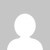# 18CSL76 Artificial Intelligence Machine Learning Laboratory

[wptelegram-join-channel]

## 18CSL76 Artificial Intelligence and Machine Learning Laboratory – VTU AIML Lab and Theory 18CS71

Artificial Intelligence and Machine Learning Laboratory – 18CSL76 (VTU AIML Lab) covers the different algorithms such as A* Search, A** Search, Find-S algorithms, Candidate elimination algorithm, Decision tree (ID3) algorithm, Artificial Neural Networks, Backpropagation Algorithm, Naïve Bayes classifier for text classification, Bayesian Belief Network, k-Means, and EM clustering Algorithm, k-Nearest Neighbour Algorithm (KNN), Locally Weighted Regression Algorithm.

1. Implement A* Search algorithm.

Implementation of A Star (*) Search Algorithm in python – Artificial Intelligence

A Star (*) Solved Numerical example – 1

2. Implement AO* Search algorithm.

Implementation of AO Star Search Algorithm in python – Artificial Intelligence

3. For a given set of training data examples stored in a .CSV file, implement and demonstrate the Candidate-Elimination algorithm to output a description of the set of all hypotheses consistent with the training examples.

Python Program to Implement Candidate Elimination Algorithm to get Consistent Version Space

Solved Numerical Examples:

Candidate Elimination Algorithm Solved Example – 1

Candidate Elimination Algorithm Solved Example – 2

Candidate Elimination Algorithm Solved Example – 3

Solved Numerical Example – Find S Algorithm to Find the Most Specific Hypothesis

Python Program to Implement FIND S Algorithm – to get Maximally Specific Hypothesis

4. Write a program to demonstrate the working of the decision tree-based ID3 algorithm. Use an appropriate data set for building the decision tree and apply this knowledge to classify a new sample.

Python Program to Implement Decision Tree ID3 Algorithm

Solved Numerical Examples and Video Tutorials on Decision Trees

How to build a decision Tree for Boolean Functions Machine Learning

How to build a decision Tree for Boolean Functions Machine Learning

How to build Decision Tree using ID3 Algorithm – Solved Numerical Example – 1

How to build Decision Tree using ID3 Algorithm – Solved Numerical Example -2

How to build Decision Tree using ID3 Algorithm – Solved Numerical Example -3

Appropriate Problems for Decision Tree Learning Machine Learning Big Data Analytics

How to find the Entropy and Information Gain in Decision Tree Learning

How to find the Entropy Given Probabilities decision Tree Learning – Machine Learning

How to find Entropy, Information Gain, Gain in terms of Gini Index, Splitting Attribute, Decision Tree – Machine Learning

How to find Entropy, Information Gain, Gini Index, Splitting Attribute, Decision Tree – Machine Learning

List and Explain Issues in Decision Tree Learning – Machine Learning

How to Avoid Overfitting in Decision Tree Learning, Machine Learning, and Data Mining

How to handle Continuous Valued Attributes in Decision Tree Learning, Machine Learning

5. Build an Artificial Neural Network by implementing the Backpropagation algorithm and test the same using appropriate data sets.

Python Program to Implement Backpropagation Algorithm

Artificial Neural Network and Backpropagation algorithm Tutorial

Appropriate Problems for Artificial Neural Networks in Machine Learning

1. Perceptron Training Rule for Linear Classification Artificial Neural Networks Machine Learning

2. AND GATE Perceptron Training Rule | Artificial Neural Networks Machine Learning

3. OR GATE Perceptron Training Rule | Artificial Neural Networks Machine Learning

Back Propagation Algorithm Artificial Neural Network Algorithm Machine Learning

Derivation of Weight Equation in Back Propagation Algorithm Artificial Neural Networks Algorithm Machine Learning

6. Write a program to implement the naïve Bayesian classifier for a sample training data set stored as a .CSV file. Compute the accuracy of the classifier, considering few test data sets.

Python Program to Implement the Naïve Bayesian Classifier

Tutorial and Solved Examples

1. Solved Example Naive Bayes Classifier to classify New Instance Naive Bayes Theorem

2. Solved Example Naive Bayes Classifier to classify New Instance Naive Bayes Theorem

3. Solved Example Naive Bayes Classifier to classify New Instance Naive Bayes Theorem

4. Solved Example Naive Bayes Classifier to classify New Instance Naive Bayes Theorem

5. Solved Example Naive Bayes Classifier to classify New Instance Naive Bayes Theorem

6. How to do Text / Document Classification using Naive Bayes Classifier and TF-IDF features

Python program to Naïve Bayesian Classifier for text classification using API

Python Program to Implement the Bayesian network using pgmpy

7. Apply EM algorithm to cluster a set of data stored in a .CSV file. Use the same data set for clustering using the k-Means algorithm. Compare the results of these two algorithms and comment on the quality of clustering. You can add Java/Python ML library classes/API in the program.

Python Program to Implement the K-Means and Estimation & MAximization (EM) Algorithm

K-Means Tutorial and Solved Examples

K Means Clustering Algorithm – Solved Numerical Example – Machine Learning

8. Write a program to implement the k-Nearest Neighbour algorithm to classify the iris data set. Print both correct and wrong predictions. Java/Python ML library classes can be used for this problem.

Python Program to Implement and Demonstrate k-Nearest Neighbour Algorithm

Tutorial and Solved Examples

Solved Numerical Example of KNN Classifier to classify New Instance IRIS Example – 1

Solved Example KNN Classifier to classify New Instance Height and Weight Example – 2

9. Implement the non-parametric Locally Weighted Regression algorithm in order to fit data points. Select the appropriate data set for your experiment and draw graphs.

Python Program to Implement and Demonstrate Locally Weighted Regression Algorithm

## Summary:

This tutorial discusses how to implement 18CSL76 Artificial Intelligence and Machine Learning Laboratory (VTU AIML Lab) experiments in python. If you like the tutorial share it with your friends. Like the Facebook page for regular updates and YouTube channel for video tutorials.

### 1 thought on “18CSL76 Artificial Intelligence Machine Learning Laboratory”

1.Dear publisher,
We are not finding the Python implementation of A* and AO* algorithms. Could you provide the same pls for AI ML Lab of new scheme of VTU.